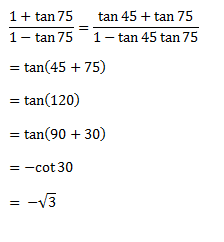# SSC CGL 2018 Practice Test Papers | Quantitative Aptitude (Day-29)

Dear Aspirants, Here we have given the Important SSC CGL Exam 2018 Practice Test Papers. Candidates those who are preparing for SSC CGL 2018 can practice these questions to get more confidence to Crack SSC CGL 2018 Examination.

[WpProQuiz 3141]

Click “Start Quiz” to attend these Questions and view Explanation

1) A father’s present age is seven years less than 30 times his son’s age 20 years ago. Also, the father’s present age is 31 years more than his son’s present age. Find the sum of their present ages, in years.

a) 75

b) 73

c) 74

d) 76

2) The speed of a body is inversely proportional to the time taken to travel a certain distance. If the speed is 40 km/hr and the time taken is 5 hrs. Find the speed if time taken is 10 hrs.

a) 80 km/hr

b) 20 km/hr

c) 60 km/hr

d) 100km/hr

3) A varies directly with the sum of B and C. B varies directly with the cube of D and `C’ varies directly with the square of D. When D = 2, A = 28, When D = 3, A = 81, what is the value of A when D = 1?

a) 176

b) 16

c) 5

d) 7

4)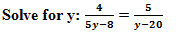a) 40/21

b) 21/40

c) -21/40

d) -40/21

5) Area of regular hexagon with side ‘a’

a) (3√3 /4) a2

b) (12/2√3 a2

c) (9/2√3) a2

d) (6/2√3) a2

6) M children are made to sit around a circle, such that every child has exactly one child sitting opposite him. If the 28th child faces the 83rd child, then find the value of M. The children are numbered from 1 to M in the clock wise direction.

a) 84

b) 220

c) 109

d) 110

7) A trader professes to sell rice at cost price, but gives 1200 gms for every 1.5 kg she sells. What are the profit percentage of the trader and the loss percentage of the customer respectively?

a) 25%, 20%

b) 20%, 25%

c) 33 1/3%, 25%

d) 25%, 33 1/3%

8) A buys a computer for Rs 30,000. He spends Rs 3,000 towards accessories. The rate of depreciation on the total cost of the computer is 20%p.a.What is his profit/loss on selling the article at rs 21,120 after three years?

a) 25%profit

b) 20%loss

c) 25%loss

d) 20%profit

9)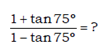a) 2/√3

b) 1/√3

c) -√3

d) √3

10) In a scalene triangle,

a) Two sides are equal

b) All three sides are equal

c) No two sides are equal

d) None of the above

Let the present age of father be f years.

Let the present age of son be s years.

F = 30 (s-20) – 7 —– (i)

f = s +31 ———— (ii)

s+31= 30 s – 600 – 7

638 = 29s

S = 22

F = 53

Sum of their ages = 22+53 = 75 years

Speed=k/time where k is a constant

40×5= k ———- (i)

Sx10=k ———- (ii)

From (i) and (ii)

S= 20 kmph

A = k (B + C)

B = m.D3

C = n. D2

A= k (m.D3+ n. D2)

When D = 2, A = 28

• 28 = k (8m + 4n) ——– (i)

When D = 3, A = 81

• 81= k (27m+9n) ——- (ii)

Solving (i) and (ii)

Km=2, kn = 3

When D=1, A= 2(1) + 3(1) = 5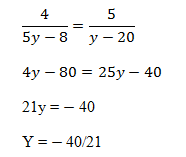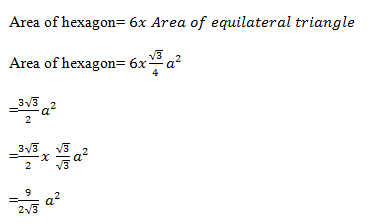Given that 28th child faces 83rd children

No. of children between 28th child and 83rd child= 83 – 28 – 1 = 54

Since they are sitting in a circle, there will be 54 children on either side

So total no. of children = 54x 2 + 2 = 110

Quantity to be sold=1500 gm

Quantity given to the customer= 1200 m

Profit= 300/1200x 100 =25%

Loss of the customer = 300/1500 x100 = 20 %

Cost price of computer = 30000+3000 = 33000

Depreciation = 20%

Cost price after 3 years=33000( 80/100)3=16896

Profit %= (21120 – 16896)/16896×100 = 25 %